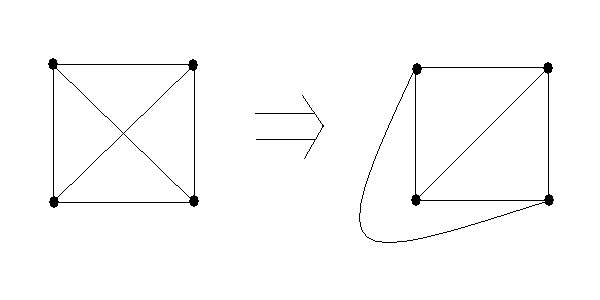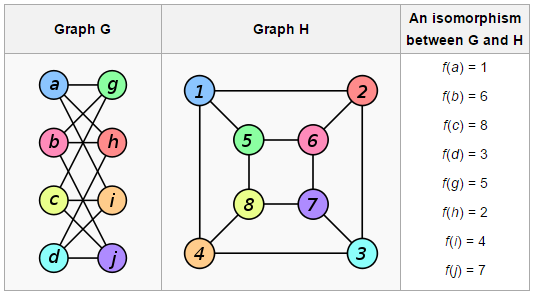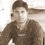# Graph Isomorphism

The graph isomorphism problem has a long history in the fields of computer science, mathematics and chemistry. Graph isomorphism is very important due to its practical applications and its unique complexity theoretic status . Informally, graph isomorphism is to check if two graphs that look different are actually the same. More formally, given two graphs does there exist a 1-to-1 mapping of vertices in one graph onto the vertices of other such that edges and non-edges are preserved?. Example of two isomorphic graphs is given belowA more interesting example is belowSome of the applications of Graph Isomorphism are verification of computer programs, Security, for example fingerprint scanners, facial scanners etc. The problem is in class NP (nondeterministic polynomial time) mean given a solution (bijective mapping) you can verify the solution efficiently.Note by Shivdutt Sharma
4 years, 7 months ago

This discussion board is a place to discuss our Daily Challenges and the math and science related to those challenges. Explanations are more than just a solution — they should explain the steps and thinking strategies that you used to obtain the solution. Comments should further the discussion of math and science.

When posting on Brilliant:

• Use the emojis to react to an explanation, whether you're congratulating a job well done , or just really confused .
• Ask specific questions about the challenge or the steps in somebody's explanation. Well-posed questions can add a lot to the discussion, but posting "I don't understand!" doesn't help anyone.
• Try to contribute something new to the discussion, whether it is an extension, generalization or other idea related to the challenge.

MarkdownAppears as
*italics* or _italics_ italics
**bold** or __bold__ bold
- bulleted- list
• bulleted
• list
1. numbered2. list
1. numbered
2. list
Note: you must add a full line of space before and after lists for them to show up correctly
paragraph 1paragraph 2

paragraph 1

paragraph 2

[example link](https://brilliant.org)example link
> This is a quote
This is a quote
    # I indented these lines
# 4 spaces, and now they show
# up as a code block.

print "hello world"
# I indented these lines
# 4 spaces, and now they show
# up as a code block.

print "hello world"
MathAppears as
Remember to wrap math in $$ ... $$ or $ ... $ to ensure proper formatting.
2 \times 3 $2 \times 3$
2^{34} $2^{34}$
a_{i-1} $a_{i-1}$
\frac{2}{3} $\frac{2}{3}$
\sqrt{2} $\sqrt{2}$
\sum_{i=1}^3 $\sum_{i=1}^3$
\sin \theta $\sin \theta$
\boxed{123} $\boxed{123}$

Sort by:

Can the graph isomorphism problem be solved in polynomial time?

- 4 years, 7 months ago

till now no one is able to solve the problem in polynomial time in general (For some special class of graphs it is solvable in polynomial time like for trees)

- 4 years, 7 months ago

Note: Laszlo Babai showed last year that Graph Isomorphism is in Quasipolynomial-time.

Staff - 4 years, 7 months ago